# Registration Errors, Terminology and Interpretation

Registration is defined as the estimation of a geometric transformation aligning objects such that the distance between corresponding points on these objects is minimized, bringing the objects into alignment.

When working with point-based registration algorithms we have three types of errors associated with our points (originally defined in ):

Fiducial Localization Error (FLE): The error in determining the location of a point which is used to estimate the transformation. The most widely used FLE model is that of a zero mean Gaussian with independent, identically distributed errors. The figure below illustrates the various possible fiducial localization errors:Fiducial Registration Error (FRE): The error of the fiducial markers following registration, $\|T(\mathbf{p_f}) - \mathbf{p_m}\|$ where $T$ is the estimated transformation and the points $\mathbf{p_f},\;\mathbf{p_m}$ were used to estimate $T$.

Target Registration Error (TRE): The error of the target fiducial markers following registration,$\|T(\mathbf{p_f}) - \mathbf{p_m}\|$ where $T$ is the estimated transformation and the points $\mathbf{p_f},\;\mathbf{p_m}$ were not used to estimate $T$.

Things to remember:

1. TRE is the only quantity of interest, but in most cases we can only estimate its distribution.
2. FRE should never be used as a surrogate for TRE as the TRE for a specific registration is uncorrelated with its FRE .
3. TRE is spatially varying.
4. A good TRE is dependent on using a good fiducial configuration .
5. The least squares solution to paired-point registration is sensitive to outliers.

 "Registration of Head Volume Images Using Implantable Fiducial Markers", C. R. Maurer Jr. et al., IEEE Trans Med Imaging, 16(4):447-462, 1997.

 "Fiducial registration error and target registration error are uncorrelated", J. Michael Fitzpatrick, SPIE Medical Imaging: Visualization, Image-Guided Procedures, and Modeling, 7261:1–12, 2009.

 "Fiducial point placement and the accuracy of point-based, rigid body registration", J. B. West et al., Neurosurgery, 48(4):810-816, 2001.

In :
import SimpleITK as sitk
import numpy as np
import copy
%matplotlib notebook
from gui import PairedPointDataManipulation, display_errors
import matplotlib.pyplot as plt
from registration_utilities import registration_errors


## FLE, FRE, TRE empirical experimentation¶

In the following cell you will use a user interface to experiment with the various concepts associated with FLE, FRE, and TRE.

### Interacting with the GUI¶

1. Change the mode and interact directly with the figure:
• Edit: Add pairs of fiducial markers with a left click and pairs of target markers with a right click.
• Markers can only be added prior to any manipulation (translation/rotation/noise/bias...)
• Translate: right-mouse button down + drag, anywhere in the figure.
• Rotate: right-mouse button down + drag, anywhere in the figure, will rotate the fiducials around their centroid
(marked by a blue dot).
2. Buttons:
• Clear Fiducials/Targets: Removes all fiducial/target marker pairs.
• Reset: Moves all marker pairs to the original locations.
• Noise: Add noise to the fiducial markers.
• Bias (FRE<TRE): Add bias to all fiducial markers so that FRE<TRE (move all markers in the same direction).
• Bias (FRE>TRE): Add bias to all fiducial markers so that FRE>TRE (move half of the markers in one direction and
the other half in the opposite direction).
• Register: Align the two point sets using the paired fiducials.

Marker glyphs:

• Light red plus: moving fiducials
• Dark red x: fixed fiducials
• Light green circle: moving targets
• Dark green square: fixed fiducials
In :
manipulation_interface = PairedPointDataManipulation(sitk.Euler2DTransform())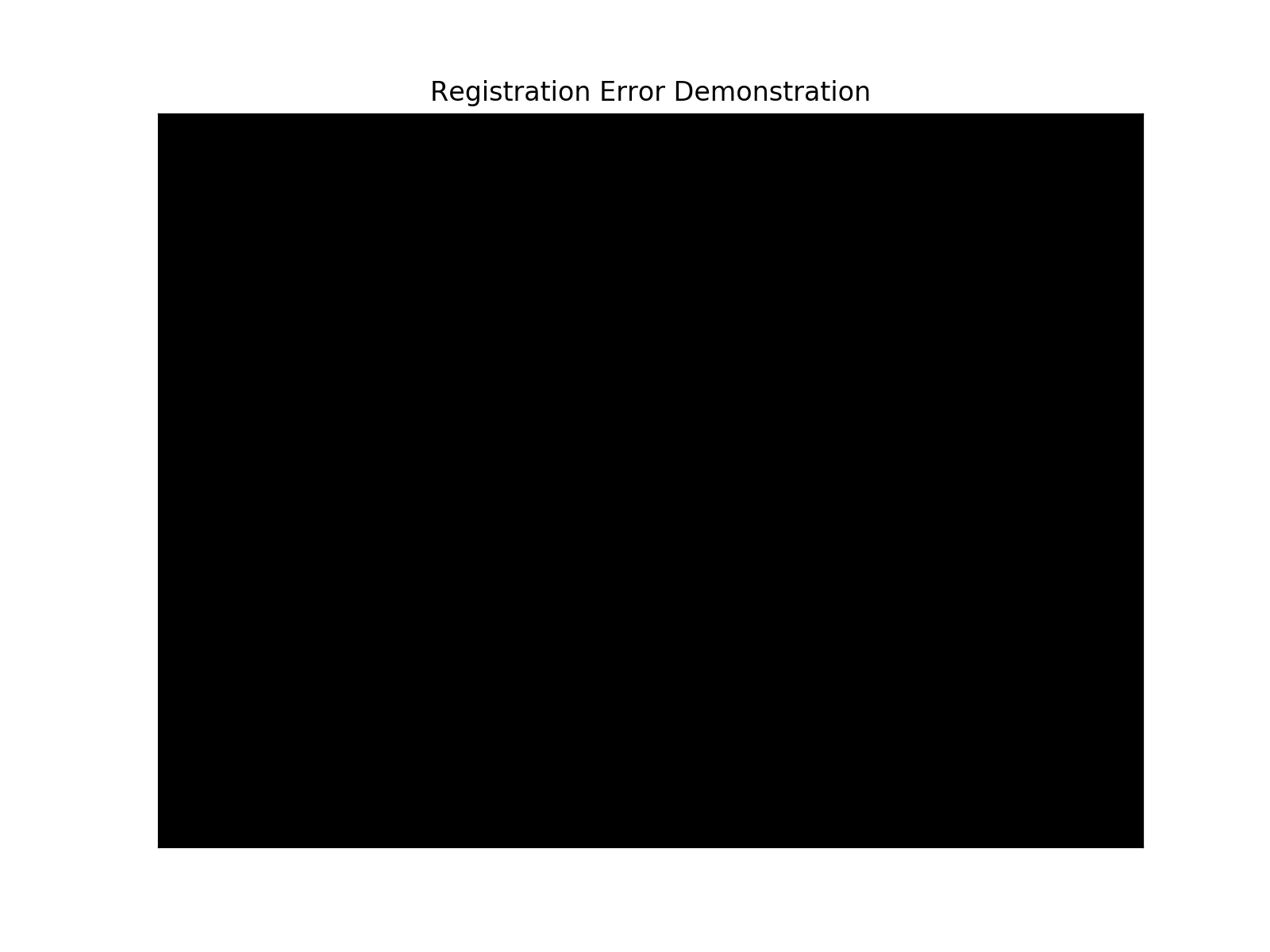## Sensitivity to outliers¶

The least-squares solutions to the paired-point registration task used by the SimpleITK LandmarkBasedTransformInitializer method are optimal under the assumption of isotropic homogeneous zero mean Gaussian noise. They will readily fail in the presence of outliers (breakdown point is 0, a single outlier can cause arbitrarily large errors).

The GUI above allows you to observe this qualitatively. In the following code cells we illustrate this quantitatively.

In :
ideal_fixed_fiducials = [[23.768817532447077, 60.082971482049849], [29.736559467930949, 68.740980140058511],
[37.639785274382561, 68.524529923608299], [41.994623984059984, 59.000720399798773]]
ideal_fixed_targets = [[32.317204629221266, 60.732322131400501], [29.413978822769653, 56.403317802396167]]
ideal_moving_fiducials = [[76.77857043206542, 30.557710579173616], [86.1401622129338, 25.76859196933914],
[86.95501792478755, 17.904506579872375], [78.07960498849866, 12.346214284259808]]
ideal_moving_targets = [[78.53588814928511, 22.166738486331596], [73.86559697098288, 24.481339720595585]]

In :
# Registration with perfect data (no noise or outliers)
fixed_fiducials = copy.deepcopy(ideal_fixed_fiducials)
fixed_targets = copy.deepcopy(ideal_fixed_targets)
moving_fiducials = copy.deepcopy(ideal_moving_fiducials)
moving_targets = copy.deepcopy(ideal_moving_targets)

# Flatten the point lists, SimpleITK expects a single list/tuple with coordinates (x1,y1,...xn,yn)
fixed_fiducials_flat = [c for p in fixed_fiducials for c in p]
moving_fiducials_flat = [c for p in moving_fiducials for c in p]

transform = sitk.LandmarkBasedTransformInitializer(sitk.Euler2DTransform(), fixed_fiducials_flat, moving_fiducials_flat)

FRE_information = registration_errors(transform, fixed_fiducials, moving_fiducials)
TRE_information = registration_errors(transform, fixed_targets, moving_targets)
FLE_values = [0.0]*len(moving_fiducials)
FLE_information =  (np.mean(FLE_values), np.std(FLE_values), np.min(FLE_values), np.max(FLE_values), FLE_values)
display_errors(fixed_fiducials, fixed_targets, FLE_information, FRE_information, TRE_information, title="Ideal Input")

# Change fourth fiducial to an outlier and register
outlier_fiducial = [88.07960498849866, 22.34621428425981]
FLE_values = np.sqrt((outlier_fiducial - moving_fiducials)**2 +
(outlier_fiducial - moving_fiducials)**2)
moving_fiducials = 88.07960498849866
moving_fiducials = 22.34621428425981

moving_fiducials_flat = [c for p in moving_fiducials for c in p]

transform = sitk.LandmarkBasedTransformInitializer(sitk.Euler2DTransform(), fixed_fiducials_flat, moving_fiducials_flat)

FRE_information = registration_errors(transform, fixed_fiducials, moving_fiducials)
TRE_information = registration_errors(transform, fixed_targets, moving_targets)
FLE_information =  (np.mean(FLE_values), np.std(FLE_values), np.min(FLE_values), np.max(FLE_values), FLE_values)
display_errors(fixed_fiducials, fixed_targets, FLE_information, FRE_information, TRE_information, title="Single Outlier")

Mean FLE 0.000000	 STD FLE 0.000000	 Min FLE 0.000000	 Max FLE 0.000000
Mean FRE 0.000000	 STD FRE 0.000000	 Min FRE 0.000000	 Max FRE 0.000000
Mean TRE 0.000000	 STD TRE 0.000000	 Min TRE 0.000000	 Max TRE 0.000000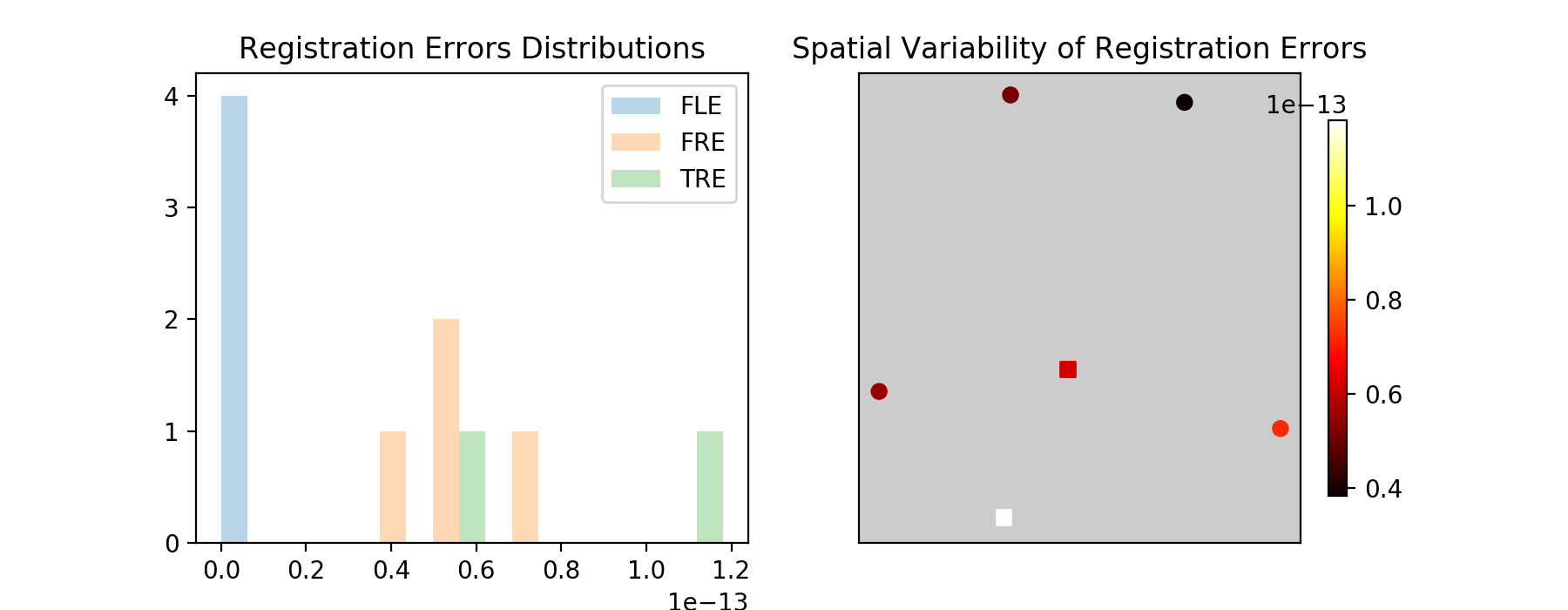Mean FLE 3.535534	 STD FLE 6.123724	 Min FLE 0.000000	 Max FLE 14.142136
Mean FRE 4.728107	 STD FRE 3.228956	 Min FRE 0.295987	 Max FRE 9.238421
Mean TRE 2.452495	 STD TRE 0.388129	 Min TRE 2.064366	 Max TRE 2.840623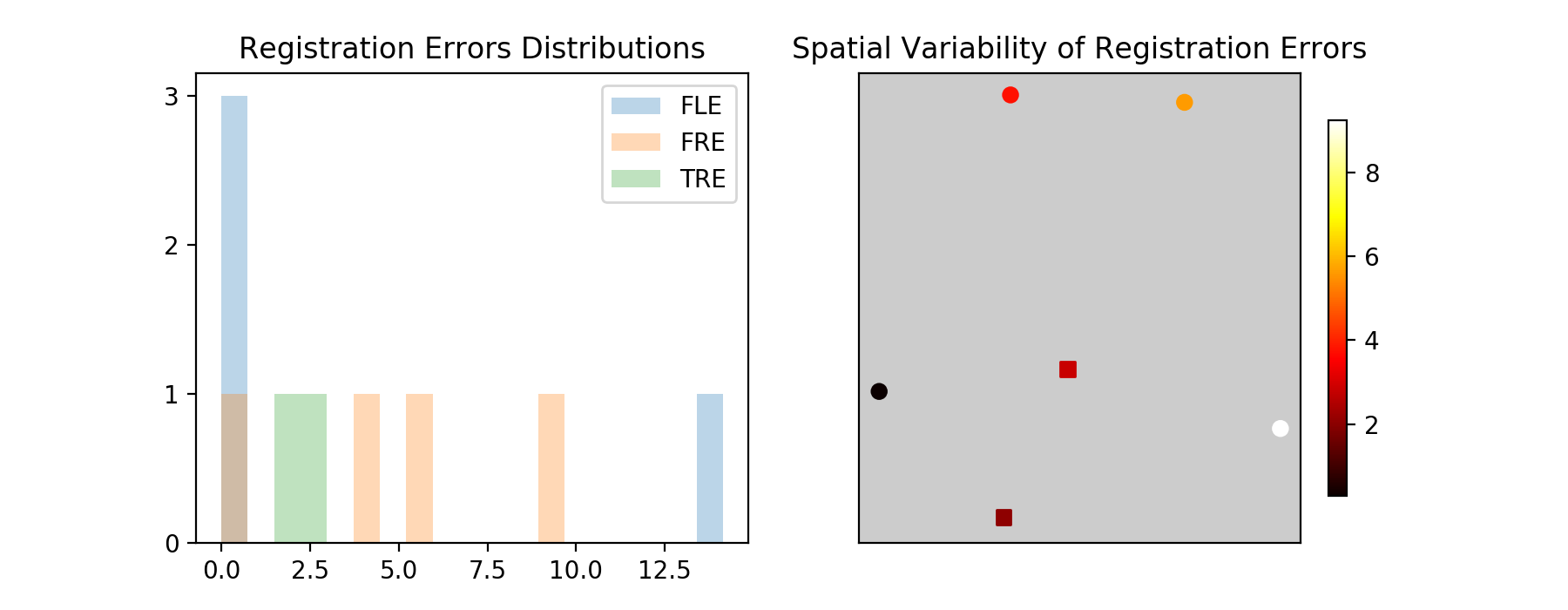## FRE is not a surrogate for TRE¶

In the next code cell we illustrate that FRE and TRE are not correlated. We first add the same fixed bias to all of the moving fiducials. This results in a large TRE, but the FRE remains zero. We then add a fixed bias to half of the moving fiducials and the negative of that bias to the other half. This results in a small TRE, but the FRE is large.

In :
# Registration with same bias added to all points
fixed_fiducials = copy.deepcopy(ideal_fixed_fiducials)
fixed_targets = copy.deepcopy(ideal_fixed_targets)
moving_fiducials = copy.deepcopy(ideal_moving_fiducials)
bias_vector = [4.5, 4.5]
bias_fle = np.sqrt(bias_vector**2 + bias_vector**2)
for fiducial in moving_fiducials:
fiducial +=bias_vector
fiducial +=bias_vector
FLE_values = [bias_fle]*len(moving_fiducials)
moving_targets = copy.deepcopy(ideal_moving_targets)

# Flatten the point lists, SimpleITK expects a single list/tuple with coordinates (x1,y1,...xn,yn)
fixed_fiducials_flat = [c for p in fixed_fiducials for c in p]
moving_fiducials_flat = [c for p in moving_fiducials for c in p]

transform = sitk.LandmarkBasedTransformInitializer(sitk.Euler2DTransform(), fixed_fiducials_flat, moving_fiducials_flat)

FRE_information = registration_errors(transform, fixed_fiducials, moving_fiducials)
TRE_information = registration_errors(transform, fixed_targets, moving_targets)
FLE_information =  (np.mean(FLE_values), np.std(FLE_values), np.min(FLE_values), np.max(FLE_values), FLE_values)
display_errors(fixed_fiducials, fixed_targets, FLE_information, FRE_information, TRE_information, title="FRE<TRE")

# Registration with bias in one direction for half the fiducials and in the opposite direction for the other half
moving_fiducials = copy.deepcopy(ideal_moving_fiducials)
pol = 1
for fiducial in moving_fiducials:
fiducial +=bias_vector*pol
fiducial +=bias_vector*pol
pol*=-1.0
FLE_values = [bias_fle]*len(moving_fiducials)
moving_targets = copy.deepcopy(ideal_moving_targets)

# Flatten the point lists, SimpleITK expects a single list/tuple with coordinates (x1,y1,...xn,yn)
fixed_fiducials_flat = [c for p in fixed_fiducials for c in p]
moving_fiducials_flat = [c for p in moving_fiducials for c in p]

transform = sitk.LandmarkBasedTransformInitializer(sitk.Euler2DTransform(), fixed_fiducials_flat, moving_fiducials_flat)

FRE_information = registration_errors(transform, fixed_fiducials, moving_fiducials)
TRE_information = registration_errors(transform, fixed_targets, moving_targets)
FLE_information =  (np.mean(FLE_values), np.std(FLE_values), np.min(FLE_values), np.max(FLE_values), FLE_values)
display_errors(fixed_fiducials, fixed_targets, FLE_information, FRE_information, TRE_information, title="FRE>TRE")

Mean FLE 6.363961	 STD FLE 0.000000	 Min FLE 6.363961	 Max FLE 6.363961
Mean FRE 0.000000	 STD FRE 0.000000	 Min FRE 0.000000	 Max FRE 0.000000
Mean TRE 6.363961	 STD TRE 0.000000	 Min TRE 6.363961	 Max TRE 6.363961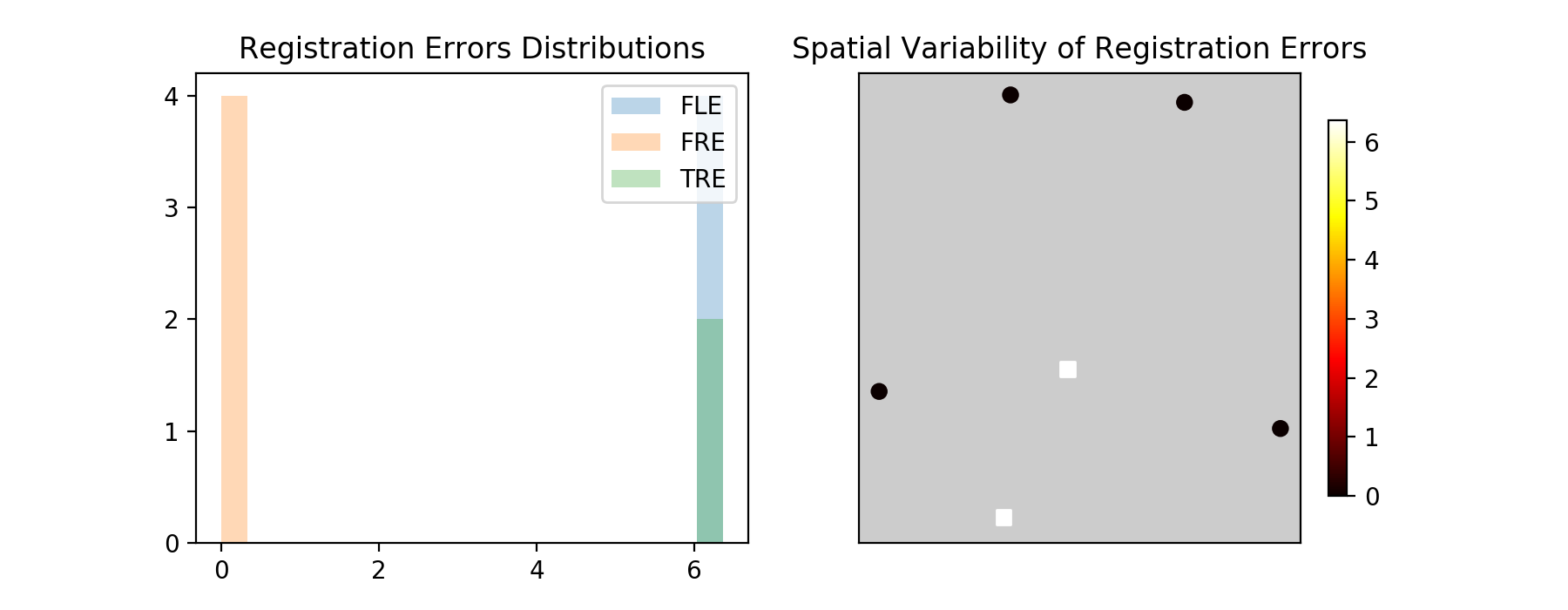Mean FLE 6.363961	 STD FLE 0.000000	 Min FLE 6.363961	 Max FLE 6.363961
Mean FRE 6.163161	 STD FRE 0.837092	 Min FRE 4.939206	 Max FRE 7.285392
Mean TRE 0.898024	 STD TRE 0.379545	 Min TRE 0.518479	 Max TRE 1.277569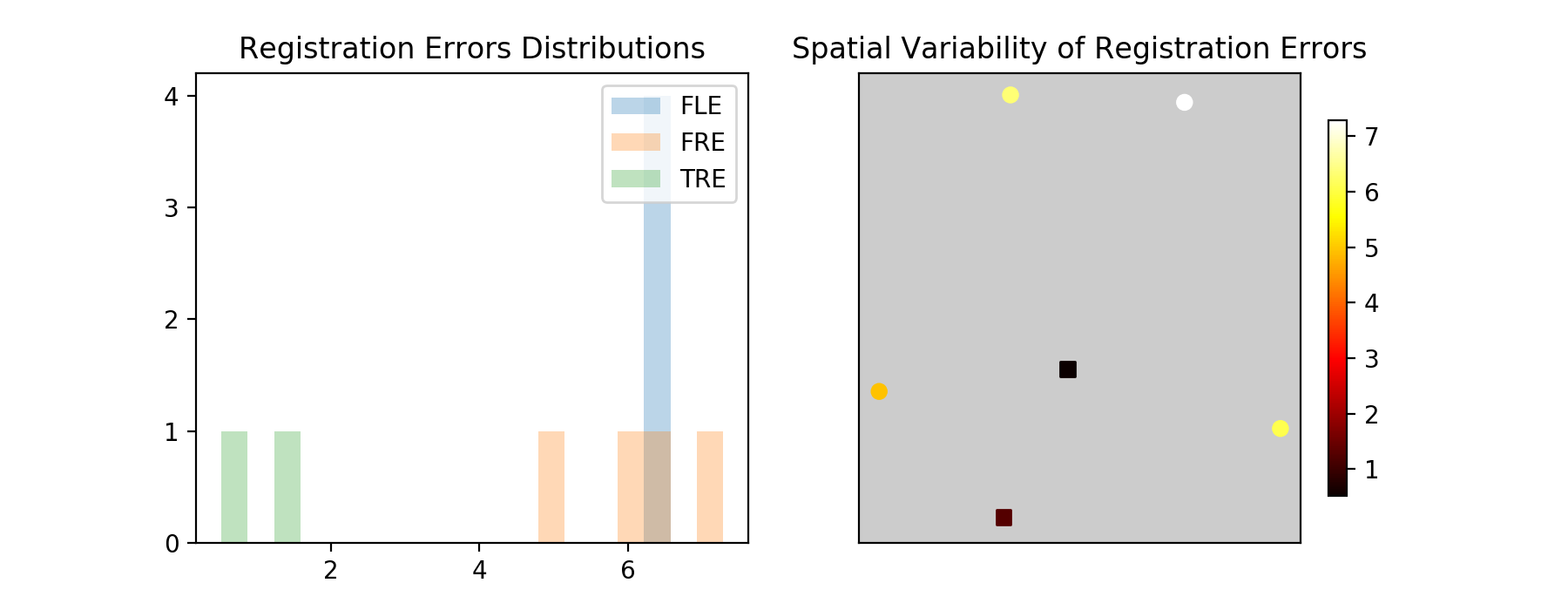## Fiducial Configuration¶

Even when our model of the world is correct, no outliers and FLE is isotropic and homogeneous, the fiducial configuration has a significant effect on the TRE. Ideally you want the targets to be at the centroid of your fiducial configuration.

This is illustrated in the code cell below. Translate, rotate and add noise to the fiducials, then register. The targets that are near the fiducials should have a better alignment than those far from the fiducials.

Now, reset the setup. Where would you add two fiducials to improve the overall TRE? Experiment with various fiducial configurations.

In :
fiducials = [[31.026882048576109, 65.696247315510021], [34.252688500189009, 70.674602293864993],
[41.349462693737394, 71.756853376116084], [47.801075596963202, 68.510100129362826],
[52.47849495180192, 63.315294934557635]]
targets = [[38.123656242124497, 64.397546016808718], [43.768817532447073, 63.748195367458059],
[26.833333661479333, 8.7698403891030861], [33.768817532447073, 8.120489739752438]]
manipulation_interface = PairedPointDataManipulation(sitk.Euler2DTransform())
manipulation_interface.set_fiducials(fiducials)
manipulation_interface.set_targets(targets)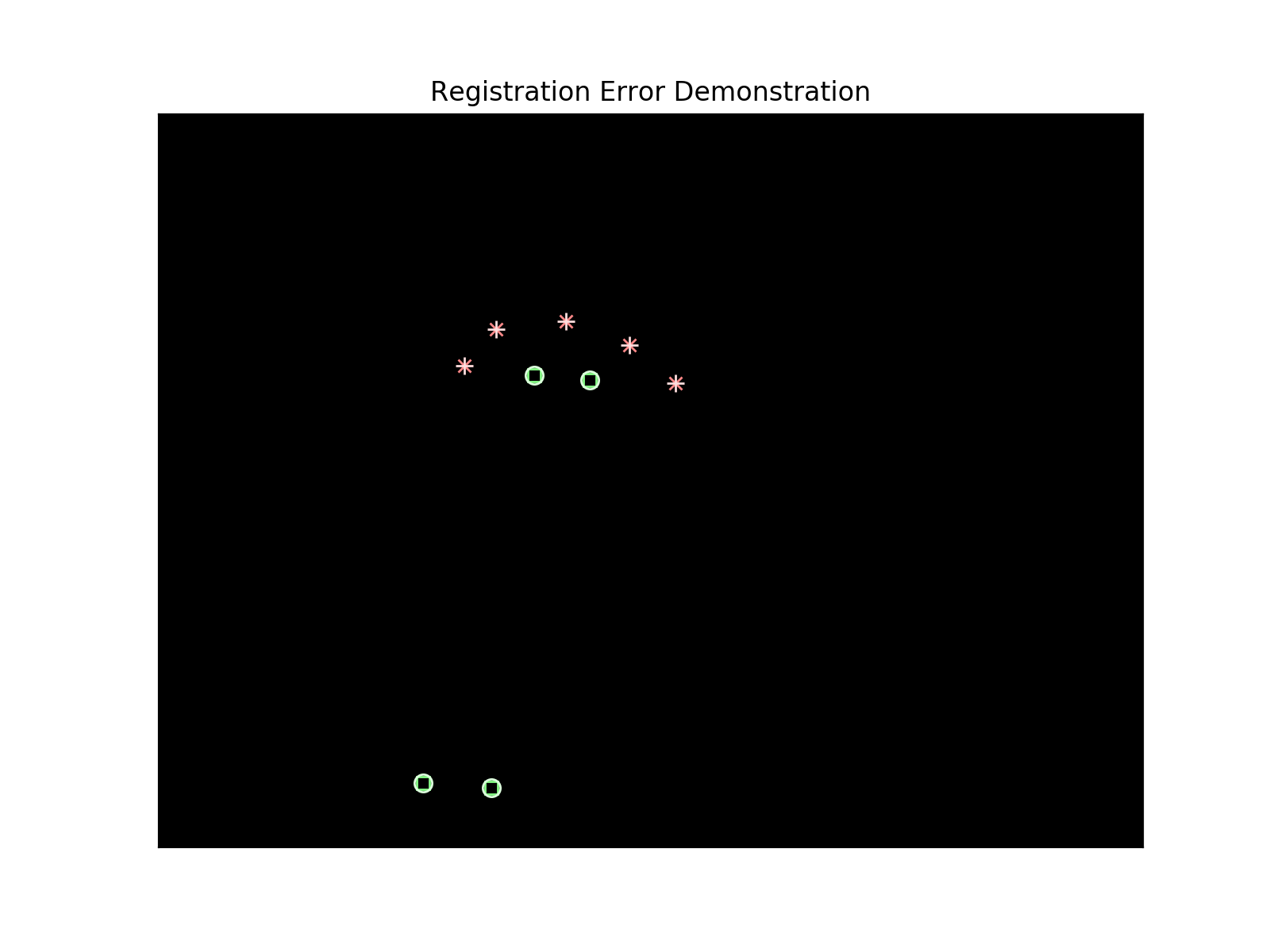## FRE-TRE, and Occam's razor¶

When we perform registration, our goal is to minimize the Target Registration Error. In practice it needs to be below a problem specific threshold for the registration to be useful.

The target point(s) can be a single point or a region in space, and we want to minimize our registration error for this target. We go about this task by minimizing another quantity, in paired-point registration this is the FRE, in the case of intensity based registration we minimize an appropriate similarity metric. In both cases we expect that TRE is minimized indirectly.

This can easily lead us astray, down the path of overfitting. In our 2D case, instead of using a rigid transformation with three degrees of freedom we may be tempted to use an affine transformation with six degrees of freedom. By introducing these additional degrees of freedom we will likely improve the FRE, but what about TRE?

In the cell below you can qualitatively evaluate the effects of overfitting. Start by adding noise with no rotation or translation and then register. Switch to an affine transformation model and see how registration effects the fiducials and targets. You can then repeat this qualitative evaluation incorporating translation/rotation and noise.

In this notebook we are working in an ideal setting, we know the appropriate transformation model is rigid. Unfortunately, this is often not the case. So which transformation model should you use? When presented with multiple competing hypotheses we select one using the principle of parsimony, often referred to as Occam's razor. Our choice is to select the simplest model that can explain our observations.

In the case of registration, the transformation model with the least degrees of freedom that reduces the TRE below the problem specific threshold.

In :
fiducials = [[31.026882048576109, 65.696247315510021],
[41.349462693737394, 71.756853376116084],
[52.47849495180192, 63.315294934557635]]

targets = [[38.123656242124497, 64.397546016808718], [43.768817532447073, 63.748195367458059]]
manipulation_interface = PairedPointDataManipulation(sitk.Euler2DTransform())
#manipulation_interface = PairedPointDataManipulation(sitk.AffineTransform(2))
manipulation_interface.set_fiducials(fiducials)
manipulation_interface.set_targets(targets)